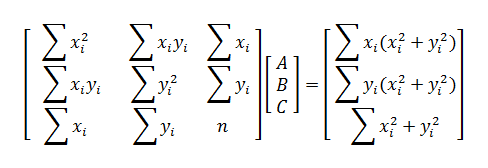# Least Squares Circle Calculator

Enter X and Y Data Pairs Below
 X Y
 X Y
 X Y

#### What Is the Best Fit Circle?

If you have (x, y) data distributed in a ring-shape on the xy-plane, you can use least squares regression to find the equation of the circle that best fits the data. That is, you determine the values of h, k, and r such that the curve

(x - h)2 + (y - k)2 = r2

provides a good fit around the data points. With least squares, "best fit" means that you minimize the equation

F(h, k, r) = ∑[(xi - h)2 + (yi - k)2 - r2]2

by solving the system ∂F/∂h = 0, ∂F/∂k = 0, and ∂F/∂r = 0. The equation of the circle can be linearized as follows:

(x - h)2 + (y - k)2 = r2
x2 - 2hx + h2 + y2 - 2ky + k2 = r2
x2 + y2 = 2hx + 2ky + r2 - h2 - k2
x2 + y2 = Ax + By + C

This equation is now linear in the undetermined coefficients A, B, and C, which means you can solve the least squares problem with matrices. After you compute A, B, and C, you can work backwards to solve for h, k, and r.

#### Finding A, B, and C with Matrices

The matrix equation for circular regression iswhere n is the number of data points (xi, yi). If the 3-by-3 matrix on the left is invertible, then there is a unique set of values for A, B, and C that generates the circle of best fit.

Once you have values for A, B, and C, you can compute h, k, and r with these transformations:

h = A/2
k = B/2
r = (√4C + A² + B²)/2

#### Example

Find the equation of the circle that best fits the five points (0, 0), (0, 5), (1, 6), (2, 7), and (8, 7).

Solving the matrix equation or using the calculator above yields

(x - 4.98735)2 + (y - 2.25605)2 = 30.572612.

If you input exactly three points into the calculator or matrix equation, you will find the equation of the circle that passes through the three given points.